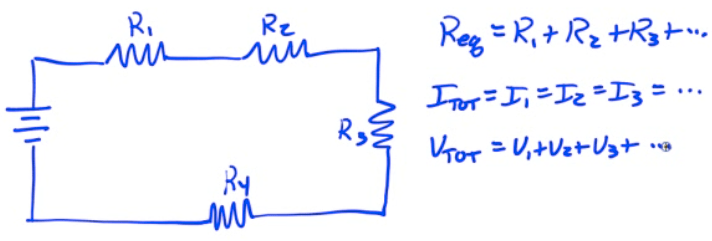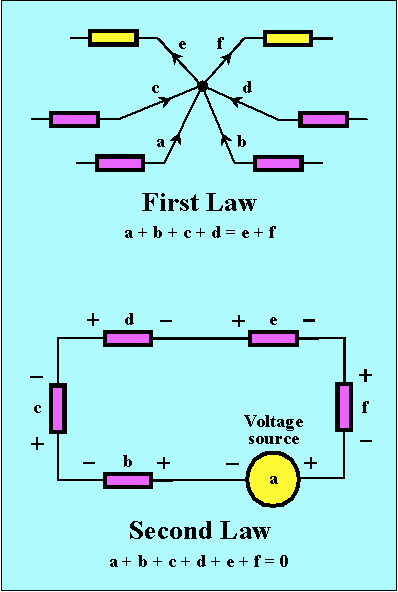# Electrical Circuits

• An electrical circuit is a closed-loop path through which current can flow

• An electrical circuit can be made up of most any materials but practically speaking, circuits are typically comprised of electrical devices

• Wires

• Batteries

• Resistors

• Switches

• Conventional current flows from high potential to low potential

# Voltmeters

• Voltmeters measure the potential difference between two points in a circuit

• Voltmeters are connected in parallel with the element to be measured

• If a voltmeter is connected correctly, you can remove it from the circuit without breaking the circuit

• Voltmeters have very high resistance# Ammeters

• Ammeters measure the current flowing through an element of a circuit

• Ammeters are connected in series with the circuit, so that the current to be measured flows through the ammeter

• The circuit must be broken to correctly insert an ammeter

• Ammeters have very low resistance to minimize the potential drop through the ammeter# Series Circuits

• Series circuits have only a single current path

• Removal of any circuit element causes an open circuit# Kirchhoff's Laws

• Kirchhoff's Laws are tools utilized in analyzing circuits

• Kirchhoff's Current Law (KCL) states that the sum of all current entering any point in a circuit equals the sum of all current leaving any point in a circuit

• Restatement of conservation of charge

• aka "Junction Rule"

• Kirchhoff's Voltage Law (KVL) states that the sum of all the potential drops in any closed loop of a circuit has to equal zero

• Restatement of conservation of energy

• aka "Loop Rule"